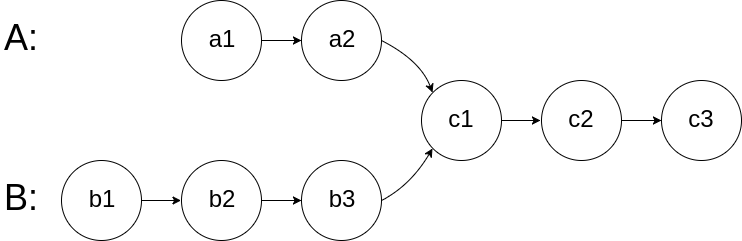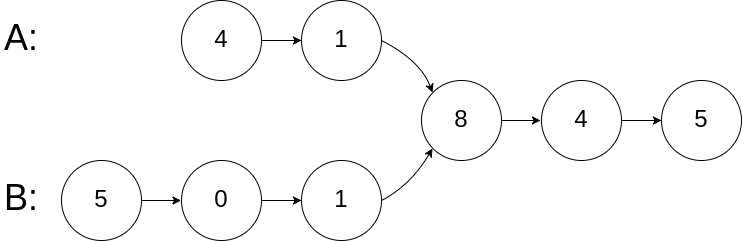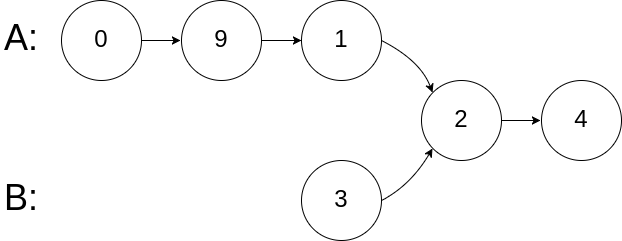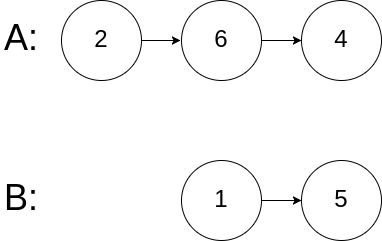# 160. Intersection of Two Linked Lists (Easy)

Write a program to find the node at which the intersection of two singly linked lists begins.

For example, the following two linked lists:begin to intersect at node c1.

Example 1:```Input: intersectVal = 8, listA = [4,1,8,4,5], listB = [5,0,1,8,4,5], skipA = 2, skipB = 3
Output: Reference of the node with value = 8
Input Explanation: The intersected node's value is 8 (note that this must not be 0 if the two lists intersect). From the head of A, it reads as [4,1,8,4,5]. From the head of B, it reads as [5,0,1,8,4,5]. There are 2 nodes before the intersected node in A; There are 3 nodes before the intersected node in B.```

Example 2:```Input: intersectVal = 2, listA = [0,9,1,2,4], listB = [3,2,4], skipA = 3, skipB = 1
Output: Reference of the node with value = 2
Input Explanation: The intersected node's value is 2 (note that this must not be 0 if the two lists intersect). From the head of A, it reads as [0,9,1,2,4]. From the head of B, it reads as [3,2,4]. There are 3 nodes before the intersected node in A; There are 1 node before the intersected node in B.
```

Example 3:```Input: intersectVal = 0, listA = [2,6,4], listB = [1,5], skipA = 3, skipB = 2
Output: null
Input Explanation: From the head of A, it reads as [2,6,4]. From the head of B, it reads as [1,5]. Since the two lists do not intersect, intersectVal must be 0, while skipA and skipB can be arbitrary values.
Explanation: The two lists do not intersect, so return null.
```

Notes:

• If the two linked lists have no intersection at all, return `null`.
• The linked lists must retain their original structure after the function returns.
• You may assume there are no cycles anywhere in the entire linked structure.
• Your code should preferably run in O(n) time and use only O(1) memory.

## Solutions

``````
class Solution {
// This problem is easy to solve. The key point is to figure out the length of both list.

// It's clear that once both list move forward simultaneous, only if they have same length
// so that they can meet at the intersect node. The trick is that we can let the longer one
// move several steps in advance before both set off. The advanced steps should be the
// difference of the longer deducting the shorter. Now they are at the same start line and
// the distance to intersect node is the same.

return null;
}

while (lenA > lenB) {
ptrA = ptrA.next;
lenA--;
}

while (lenB > lenA) {
ptrB = ptrB.next;
lenB--;
}

while (ptrA != null && ptrB != null) {
if (ptrA == ptrB) {
return ptrB;
}

ptrA = ptrA.next;
ptrB = ptrB.next;
}

return null;
}

int l = 0;

l++;
}

return l;
}
}
``````